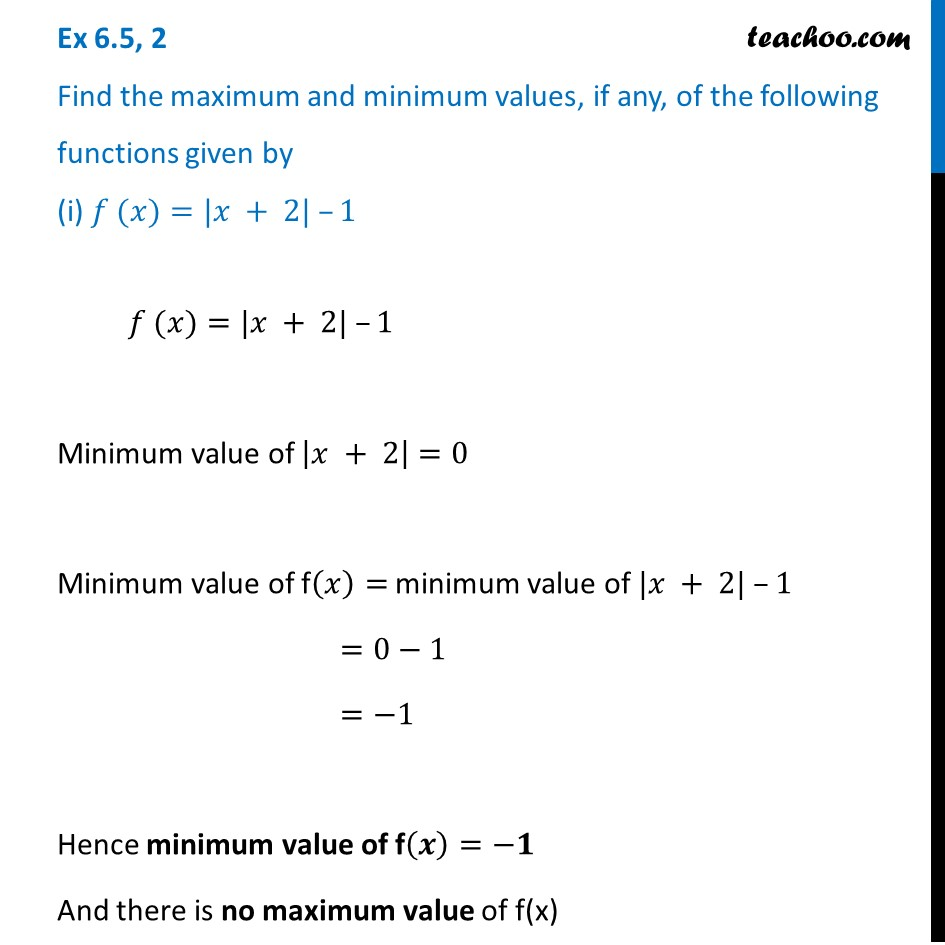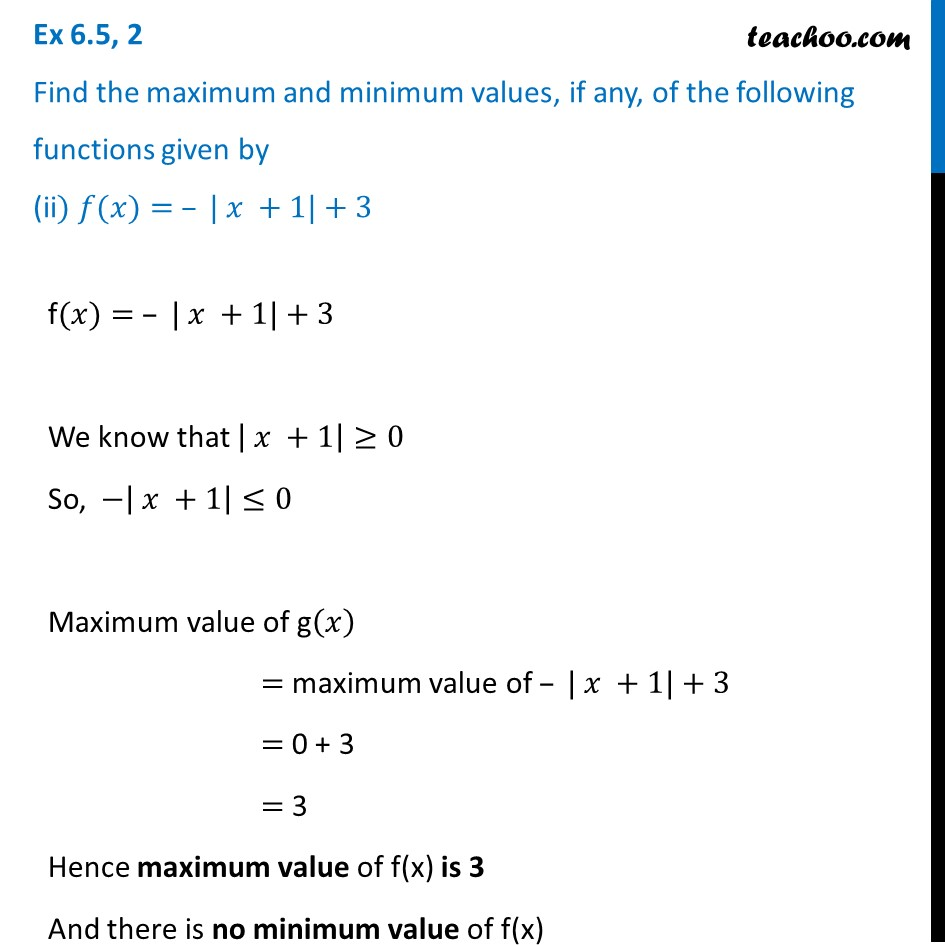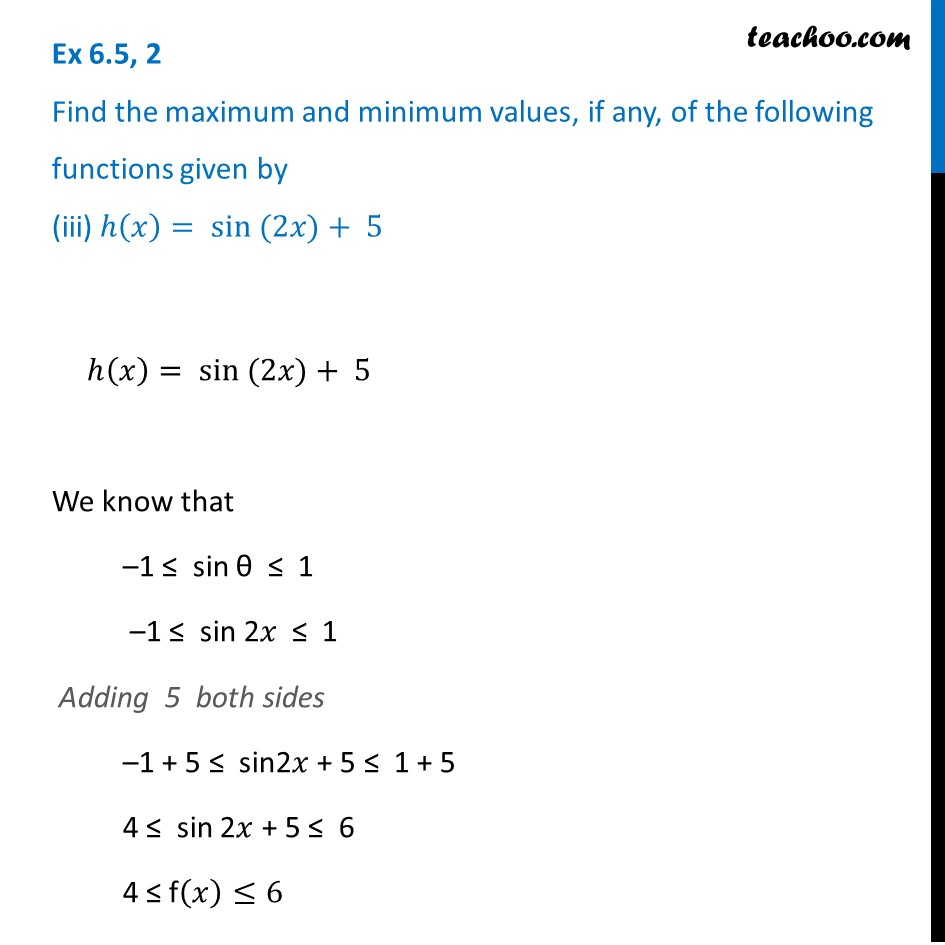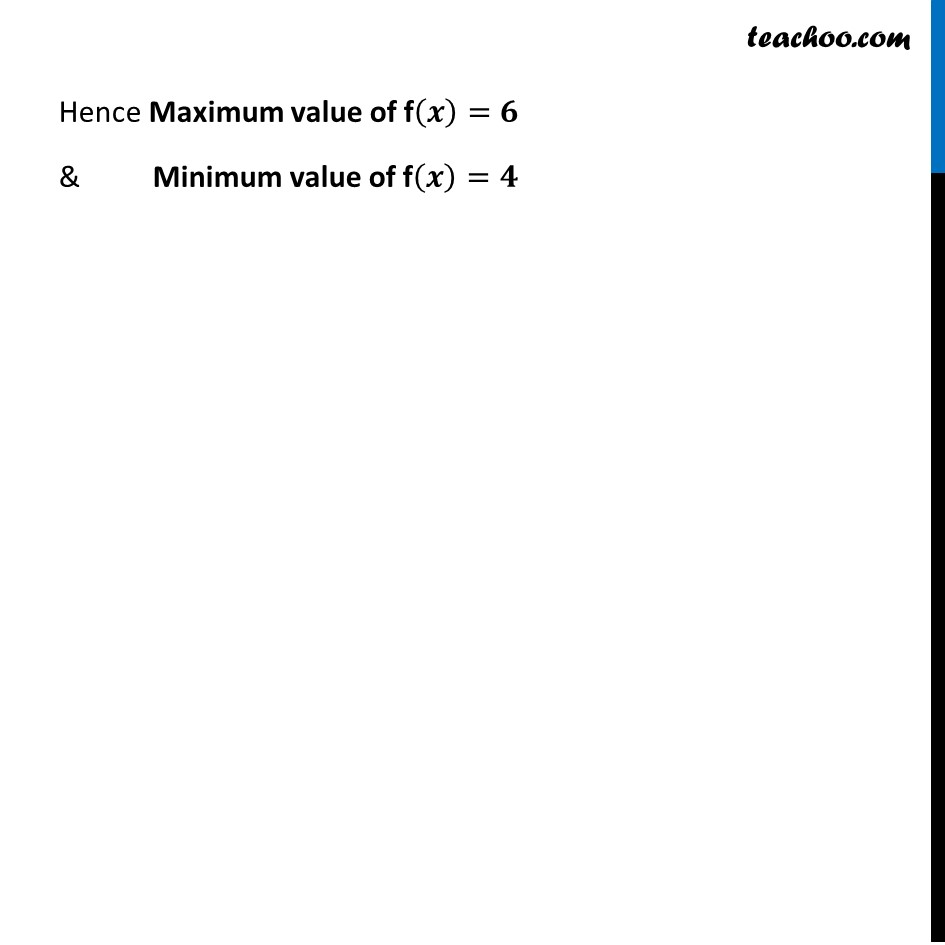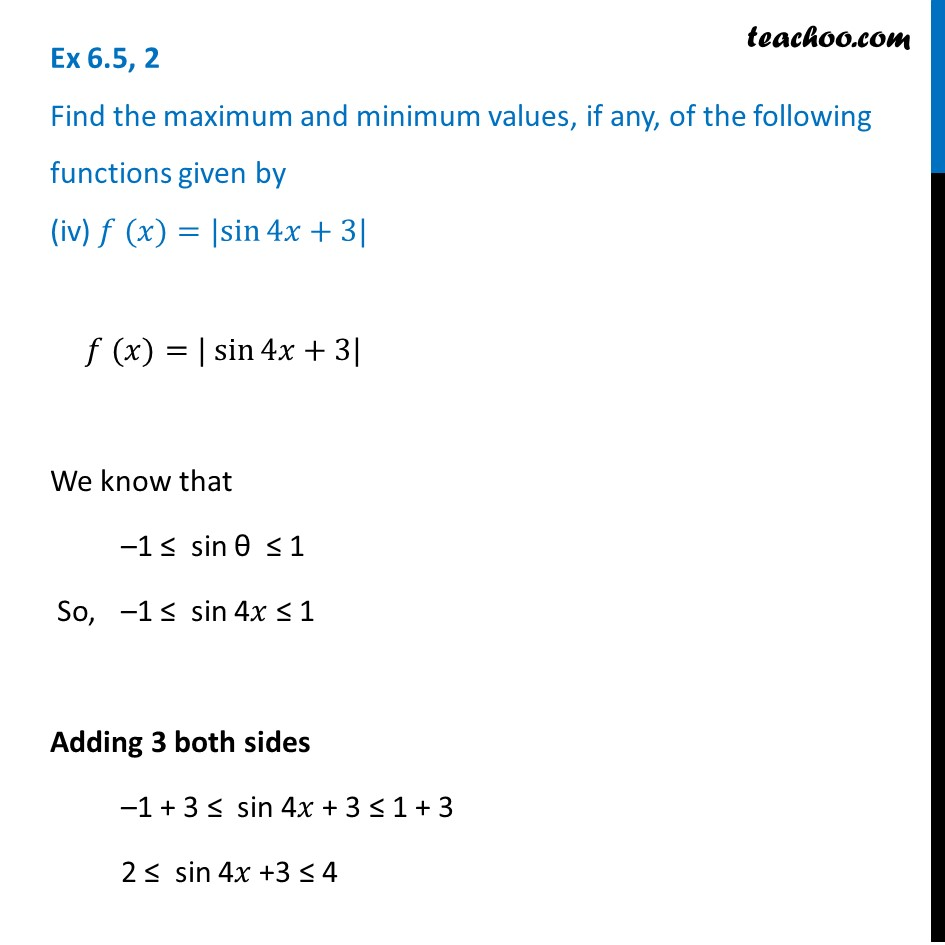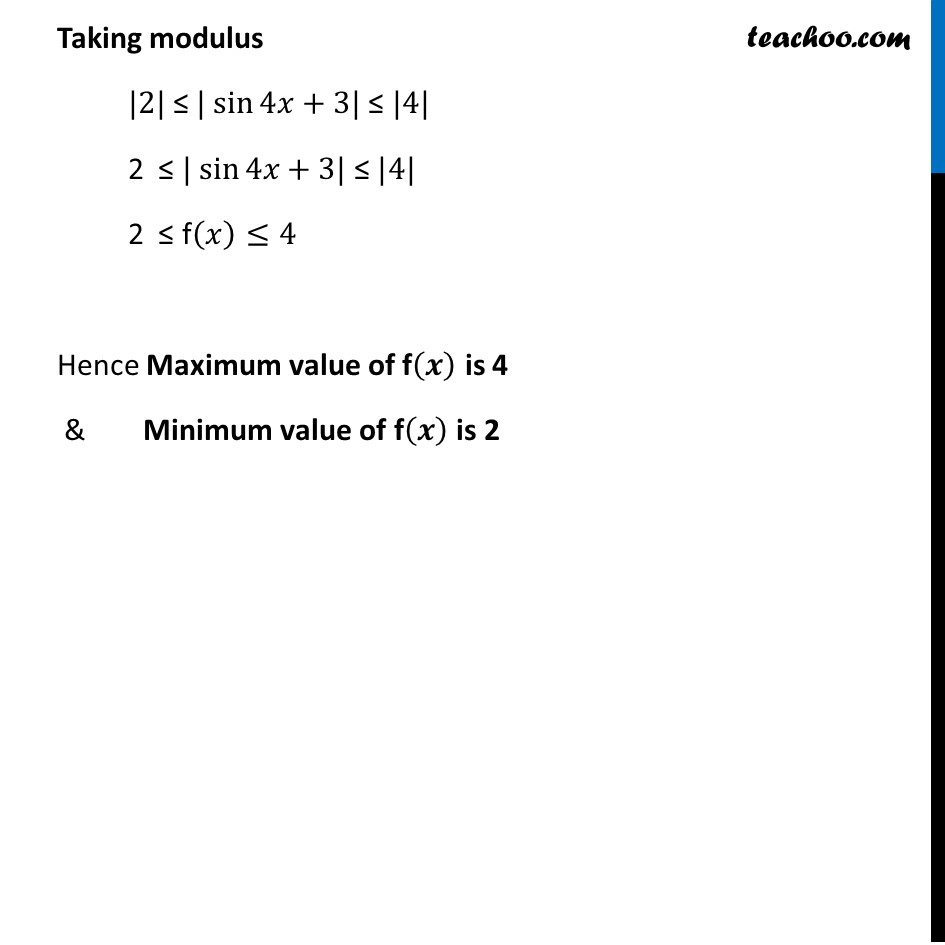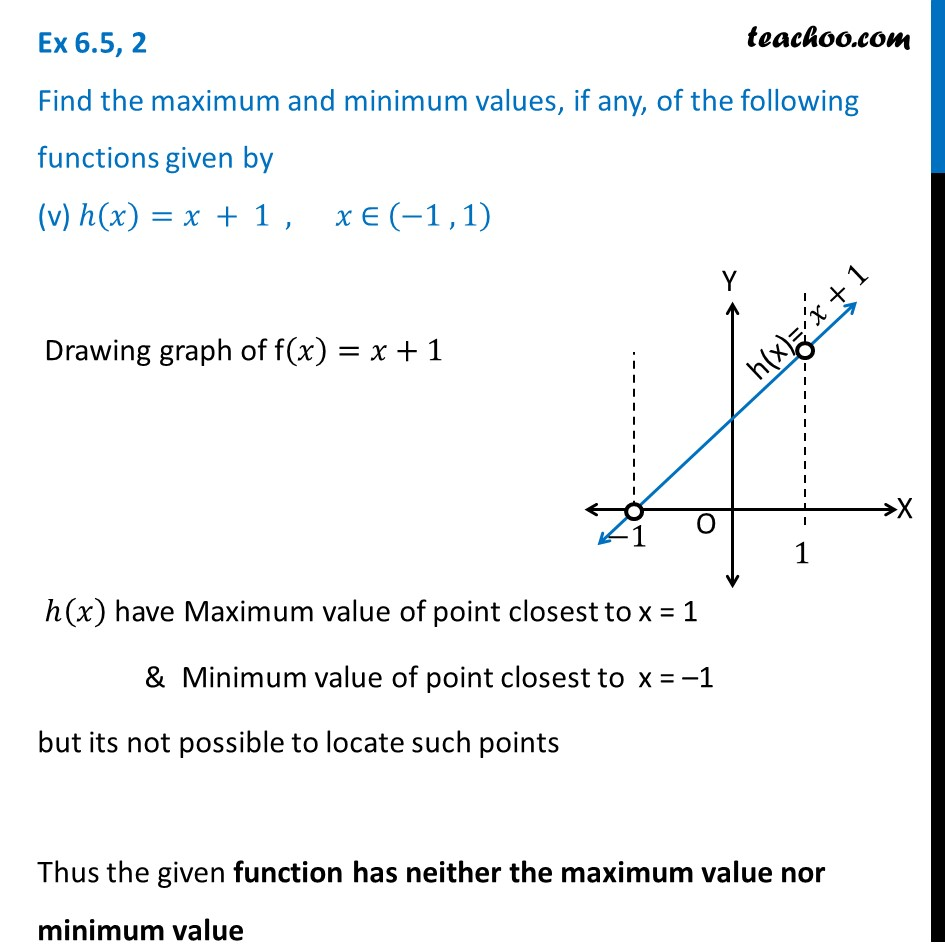1. Chapter 6 Class 12 Application of Derivatives
2. Serial order wise
3. Ex 6.5

Transcript

Ex 6.5, 2 Find the maximum and minimum values, if any, of the following functions given by (i) 𝑓 (𝑥)=|𝑥 + 2| –1 𝑓 (𝑥)=|𝑥 + 2| –1 Minimum value of |𝑥 + 2|=0 Minimum value of f(𝑥)= minimum value of |𝑥 + 2| –1 =0−1 =−1 Hence minimum value of f(𝒙)=−𝟏 And there is no maximum value of f(x) Ex 6.5, 2 Find the maximum and minimum values, if any, of the following functions given by (ii) 𝑓(𝑥)= – | 𝑥 +1|+3f(𝑥)= – | 𝑥 +1|+3 We know that | 𝑥 +1|≥0 So, −| 𝑥 +1|≤0 Maximum value of g(𝑥) = maximum value of – | 𝑥 +1|+3 = 0 + 3 = 3 Hence maximum value of f(x) is 3 And there is no minimum value of f(x) Ex 6.5, 2 Find the maximum and minimum values, if any, of the following functions given by (iii) ℎ(𝑥)= sin ⁡(2𝑥)+ 5ℎ(𝑥)= sin ⁡(2𝑥)+ 5 We know that –1 ≤ sin θ ≤ 1 –1 ≤ sin 2𝑥 ≤ 1 Adding 5 both sides –1 + 5 ≤ sin2𝑥 + 5 ≤ 1 + 5 4 ≤ sin 2𝑥 + 5 ≤ 6 4 ≤ f(𝑥)≤6 Hence Maximum value of f(𝒙)=𝟔 & Minimum value of f(𝒙)=𝟒 Ex 6.5, 2 Find the maximum and minimum values, if any, of the following functions given by (iv) 𝑓 (𝑥)=|sin⁡4𝑥+3|𝑓 (𝑥)=| sin⁡4𝑥+3| We know that –1 ≤ sin θ ≤ 1 So, –1 ≤ sin 4𝑥 ≤ 1 Adding 3 both sides –1 + 3 ≤ sin 4𝑥 + 3 ≤ 1 + 3 2 ≤ sin 4𝑥 +3 ≤ 4 Taking modulus |2| ≤ | sin⁡4𝑥+3| ≤ |4| 2 ≤ | sin⁡4𝑥+3| ≤ |4| 2 ≤ f(𝑥)≤4 Hence Maximum value of f(𝒙) is 4 & Minimum value of f(𝒙) is 2 Ex 6.5, 2 Find the maximum and minimum values, if any, of the following functions given by (v) ℎ(𝑥)=𝑥 + 1 , 𝑥 ∈ (−1 , 1) Drawing graph of f(𝑥)=𝑥+1 ℎ(𝑥) have Maximum value of point closest to x = 1 & Minimum value of point closest to x = –1 but its not possible to locate such points Thus the given function has neither the maximum value nor minimum value

Ex 6.5